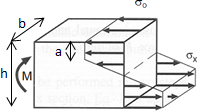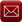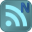You have downloaded this file 0 times in the last 24 hours, limit is 100.

## Residual Stress.xls This location is for Registered Users Only.Perhaps you need to login or register.

Description:Residual Stress Calculation
Purpose of calculation
Calculate residual stress in a rectangular cross section when a moment is applied.

Calculation Reference
First Principles

Calculation Validation
Use XLC add-in to check cell formulas
Check that elastic moment calculated by two methods are equal.

Any Consistent Calculation Units

Section inertia and moment require to reach material elastic limit
Depth of plastic zone
Elastic moment calculated by elastic stress over area of section
Plastic moment calculated by plastic stress over area of section
Plasticity factor on elastic moment

Calculation Reference
Residual Stress
Formulas for stress and strain
Stress analysis

Submitted By:
Submitted On:
19 Jul 2011
File Size:
364.50 Kb
44
File Version:
1.0
File Author:
John Doyle
Rating:Total Votes:1Email (preferred method of contact)US +1 617 5008224EU +44 113 8152220